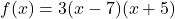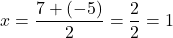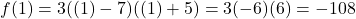## Find the vertex of f(x) = 3(x-7)(x+5) Please show your work. ^^ I’ve been having a bit of trouble with this question :( a

Question

Find the vertex of f(x) = 3(x-7)(x+5)

^^ I’ve been having a bit of trouble with this question 🙁 although it’s simple quadratics is not my strong suit

in progress 0
7 months 2021-07-23T08:56:06+00:00 2 Answers 8 views 0

## Answers ( )

vertex (1, -108)

Step-by-step explanation:

First find the zeros

f(x) = 3(x-7)(x+5)

0 =  3(x-7)(x+5)

Using the zero product property

x-7 = 0   x+5 = 0

x = 7  x = -5

The x coordinate of the vertex is the average of the zeros

(7+-5)/2 = 2/2 =1

To find the y coordinate, substitute the x coordinate into the equation

y = 3(1-7)(1+5) = 3(-6)(6) = -108

The vertex is at (1, -108).

Step-by-step explanation:

We have the function:And we want to find its vertex point.

Note that this is in factored form. Hence, our roots/zeros are x = 7 and x = -5.

Since a parabola is symmetric along its vertex, the x-coordinate of the vertex is halfway between the two zeros. Hence:To find the y-coordinate, substitute this back into the function. Hence:Therefore, our vertex is at (1, -108).